Miscellaneous

Chapter 5 Class 11 Complex Numbers
Serial order wise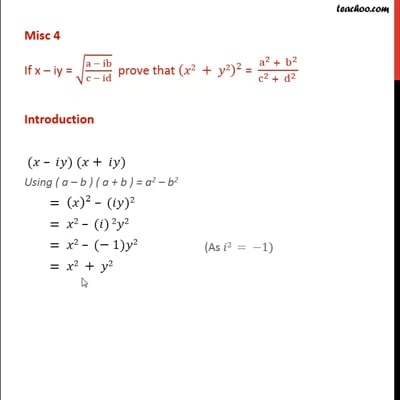This video is only available for Teachoo black users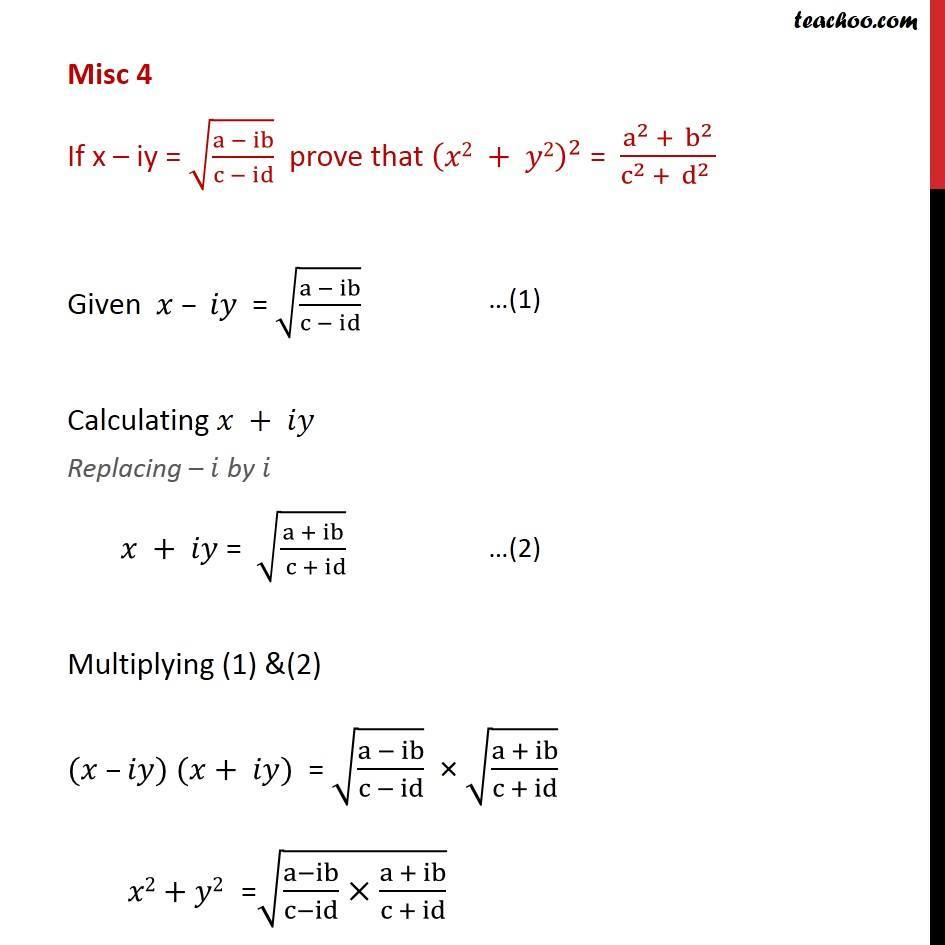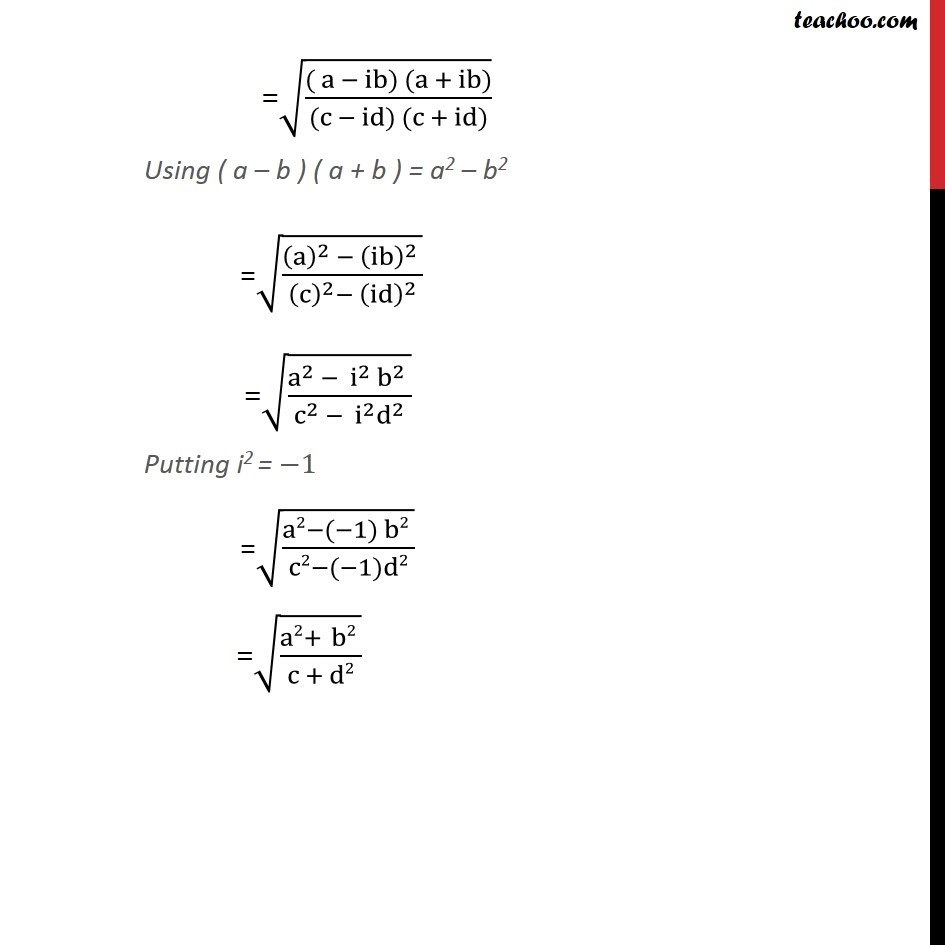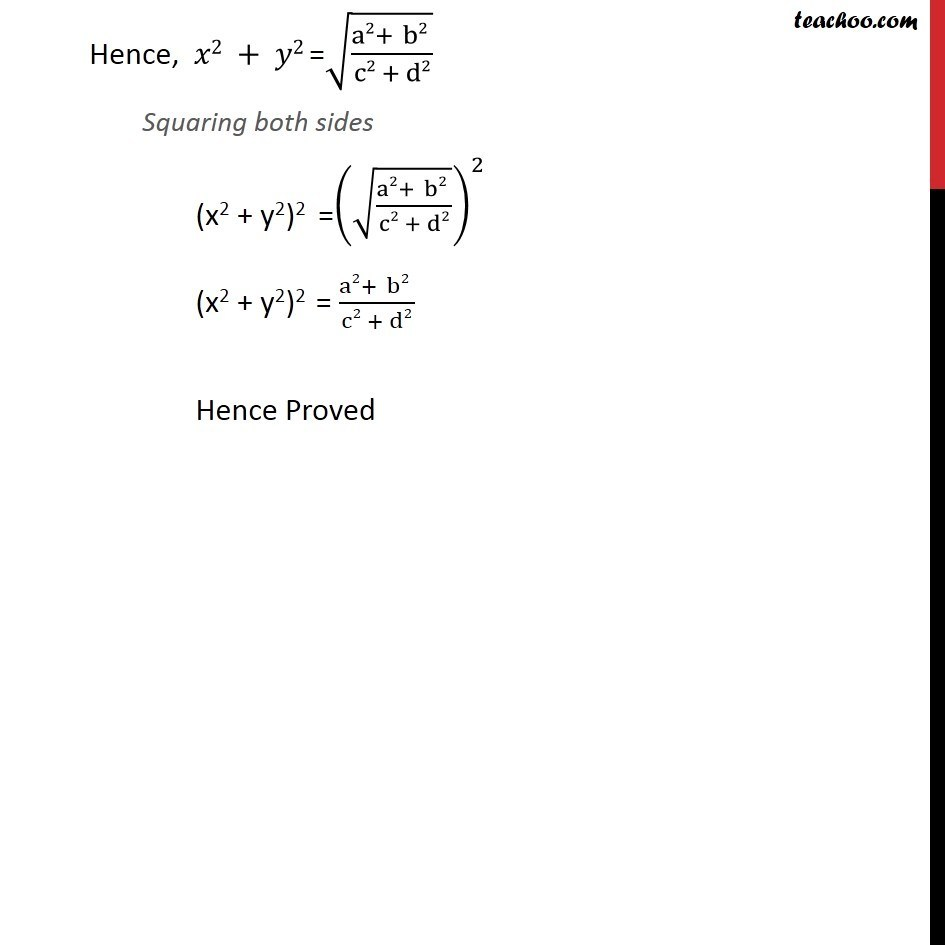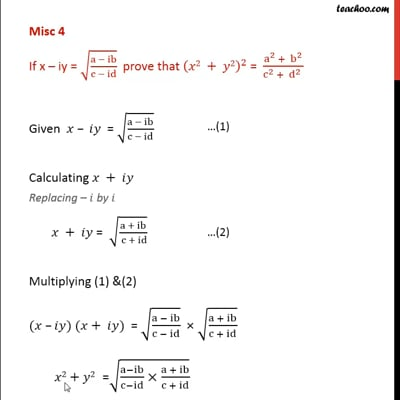This video is only available for Teachoo black users

Solve all your doubts with Teachoo Black (new monthly pack available now!)

### Transcript

Misc 4 If x – iy = √((a − ib)/(c − id)) prove that (𝑥2 + 𝑦2)^2 = (a^2 + b^2)/(c^2 + d^2 ) Introduction (𝑥 – 𝑖𝑦) (𝑥+ 𝑖𝑦) Using ( a – b ) ( a + b ) = a2 – b2 = (𝑥)^2 – (𝑖𝑦)2 = 𝑥2 – (𝑖) 2𝑦2 = 𝑥2 – (− 1)𝑦2 = 𝑥2 + 𝑦2 Misc 4 If x – iy = √((a − ib)/(c − id)) prove that (𝑥2 + 𝑦2)^2 = (a^2 + b^2)/(c^2 + d^2 ) Given 𝑥 – 𝑖𝑦 = √((a − ib)/(c − id)) Calculating 𝑥 + 𝑖𝑦 Replacing – 𝑖 by 𝑖 𝑥 + 𝑖𝑦 = √((a + ib)/( c + id)) Multiplying (1) &(2) (𝑥 –𝑖𝑦) (𝑥+ 𝑖𝑦) = √((a − ib)/(c − id)) × √((a + ib)/(c + id)) 𝑥2+𝑦2 =√((a−ib)/(c−id)×(a + ib)/(c + id)) =√((( a − ib) (a + ib))/((c − id) (c + id))) Using ( a – b ) ( a + b ) = a2 – b2 =√(((a)^2 − (ib)^2 )/((c)^2−〖 (id)〗^2 )) =√((a^2 − i^2 b^2 )/(c^2 − i^2 d^2 )) Putting i2 = −1 =√((a2−(−1) b2 )/(c2−(−1)d2)) =√((a2+ b2 )/(c + d2)) Hence, 𝑥2 + 𝑦2 =√((a2+ b2 )/(c2 + d2)) Squaring both sides (x2 + y2)2 =(√((a2+ b2 )/(c2 + d2)))^2 (x2 + y2)2 = (a2+ b2 )/(c2 + d2) Hence Proved